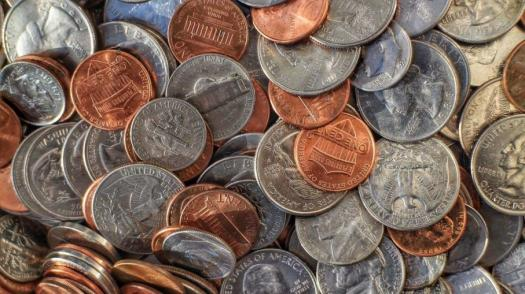# The Coin Problem Quiz

10 Questions | Total Attempts: 132SettingsWe all need to learn how to handle our money, and that comes with knowing the value of a certain coin to the dollar. We may not end up becoming financial geniuses one day but we need to know the value of the very money we work so hard for. So, do you think you can solve our coin problem here? Take our quiz and find out.

• 1.
Josh has 4 more quarters than dimes. If he has a total of \$1.70, how many quarters and dimes does he have?
• A.

2

• B.

3

• C.

2.5

• D.

3.5

• 2.
Chantel has \$4.85 in coins. If she has 6 more nickels than dimes and twice as many quarters as dimes, how many coins of each type does she have?
• A.

5

• B.

7

• C.

10

• D.

6

• 3.
Tarzan bought a pencil for Jane and received change for \$3 and 20 coins, all nickels and quarters. How many of each kind are given?
• A.

6 nickels and 10 quarters

• B.

15 nickels and 10 quarters

• C.

5 nickels and 5 quarters

• D.

10 nickels and 10 quarters

• 4.
Magalia received a money order worth \$13. She received 10 more dimes than nickels, and 22 more quarters than dimes. How many coins of each did she receive?
• A.

5 nickels, 20 dimes, and 40 quarters.

• B.

20 dimes, and 42 quarters.

• C.

10 nickels, 20 dimes, and 42 quarters.

• D.

10 nickels, 20 dimes, and 2 quarters.

• 5.
John has a total of 19 nickels and dimes worth \$1.65. How many of each type of coin does he have?
• A.

5 nickels and 14 dimes

• B.

5 nickels and 10 dimes

• C.

5 nickels and 12 dimes

• D.

2 nickels and 14 dimes

• 6.
Paloma has quarters and nickels. She has twice as many quarters as nickels. If the value of the coins totals \$4.40, how many quarters and nickels does Paloma have?
• A.

8 nickels and 16 quarters

• B.

4 nickels and 16 quarters

• C.

8 nickels and 10 quarters

• D.

18 nickels and 16 quarters

• 7.
Mom had \$11.85 in her purse. She only had nickels and quarters in her purse. The number of quarters is equal to one less than twice the number of nickels. How many of each kind of coin did she have?
• A.

10 nickels and 43 quarters

• B.

20 nickels and 43 quarters

• C.

22 nickels and 43 quarters

• D.

22 nickels and 40 quarters

• 8.
Grandma saves all of her quarters and dimes in a jar. She collected 170 coins that are worth \$26. How many of each coin does she have?
• A.

60 quarters and 110 dimes

• B.

40 quarters and 110 dimes

• C.

60 quarters and 100 dimes

• D.

50 quarters and 90 dimes

• 9.
Colin has \$1.15 in his wallet, which contains 18 coins in nickels and dimes. Find how many of each kind coin he has in his wallet.
• A.

5 nickels and 5 dimes

• B.

13 nickels and 5 dimes

• C.

5 nickels and 115 dimes

• D.

13 nickels and 8 dimes

• 10.
In a collection of dimes and quarters there are 6 more dimes than quarters. If there is \$29.65 overall, how many of each are there?
• A.

183 quarters and 89 dimes

• B.

83 quarters and 89 dimes

• C.

83 quarters and 189 dimes

• D.

3 quarters and 89 dimesBack to top